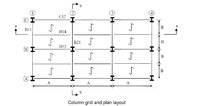# Analysis of column load question for a concrete floor

#### JimCrown

##### New member
I am struggling to get my head around the analysis of column load question for a concrete floor. I feel like I have worked out all the design loads. But, I am not sure if they are correct? Am I going down the right path or is this right?

The figure below shows the column grid and the plan layout of the steel beams for the floor of a 3 storey building. All beams may be assumed to be simply supported at their ends.

A = 6500 mm
B = 3500 mmQ1) The beams support a 215 mm thick, precast concrete floor, spanning (one way) in the direction shown in the diagram. Perimeter beams also support external cladding in the form of one lead of 100 mm thick, lightweight concrete blockwork, 3.66m high (the storey height of the building). Imposed loading on the floor is 2.5 kn/m^2 (characteristic value).

Determine the design loads at the serviceability SLS and ultimate limit state ULS for beams C12, B14, B12, B11 and B21.

NOTE: use Eurocode 1 to find the density of concrete (normal weight). This would be 25 KN/M^3

Use of density of blockwork = 13 Kn/m^3. Self-weight of the steel beams may be neglected. Prior to carry out the analysis, you are advised to consider the units of the loads determined in Q1 and convert them accordingly.

Concrete Slab thickness - 215 mm
Imposed load on slab - 2.5 kn/m^2

Calculations

External Cladding - C12 - 13 x (100/1000) x 3.66 x (6500/1000) = 30.927 kn
(M/6.5) = (30.927/6.5) = 4.758 kn/m

External Cladding - B11 - 13 x (100/1000) x 3.66 x (3500/1000) = 16.653 kn
(M/3.5) = (16.653 /3.5) = 4.758 kn/m

Concrete slab self - weight - 0.215 x 25 = 5.375 kn/m^2
Total self-weight of slab + external cladding = 5.375 + 4.758 = 10.133 kn/m^2

Beam C12 - UD Loads on Beam C12

Concrete Slab + External Cladding - 10.133 x 6.5 x 1.75 = 115.262875 kn (Dead Load)
Imposed Load - 2.5 x 6.5 x 1.75 = 28.4375 kn (Imposed Load)

UD Load Serviceability Limit State

115.262875 x 1 + 28.4375 x 1 = 143.700375 kn

UD Load Ultimate Limit State

115.262875 x 1.35 + 28.4375 x 1.5 = 198.2611313 kn

Beam C12 isolated

Reaction A - (Serv) - 143.700375 / 2 = 71.8501875 kn
Reaction A - (Ult) - 198.2611313 / 2 = 99.13056563 kn

Reaction B - (Serv) - 143.700375/ 2 = 71.8501875 kn
Reaction B - (Ult) - 198.2611313 / 2 = 99.13056563 kn

Beam B14 - UD Loads on Beam B14

Concrete Slab - 5.375 x 6.5 x 1.75 = 61.140625 kn (Dead Load)
Imposed Load - 2.5 x 6.5 x 1.75 = 28.4375 kn (Imposed Load)

UD Load Serviceability Limit State

61.140625 x 1 + 28.4375 x 1 = 89.578125 kn

UD Load Ultimate Limit State

61.140625 x 1.35 + 28.4375 x 1.5 = 125.1960938 kn

Beam B14 isolated

Reaction A - (Serv) - 89.578125 / 2 = 44.7890625 kn
Reaction A - (Ult) - 125.1960938 / 2 = 62.5980469 kn

Reaction B - (Serv) - 89.578125 / 2 = 44.7890625 kn
Reaction B - (Ult) - 125.1960938 / 2 = 62.5980469 kn

Beam B12 - UD Loads on Beam B12

Concrete Slab - 5.375 x 6.5 x 1.75 = 61.140625 kn (Dead Load)
Imposed Load - 2.5 x 6.5 x 1.75 = 28.4375 kn (Imposed Load)

UD Load Serviceability Limit State

61.140625 x 1 + 28.4375 x 1 = 89.578125 kn

UD Load Ultimate Limit State

61.140625 x 1.35 + 28.4375 x 1.5 = 125.1960938 kn

Beam B12 isolated

Reaction A - (Serv) - 89.578125 / 2 = 44.7890625 kn
Reaction A - (Ult) - 125.1960938 / 2 = 62.5980469 kn

Reaction B - (Serv) - 89.578125 / 2 = 44.7890625 kn
Reaction B - (Ult) - 125.1960938 / 2 = 62.5980469 kn

Beam B11 - UD Loads on Beam B11

External Cladding - 4.758 x 7.0 = 33.306 kn (Dead Load)

UD Load Serviceability Limit State

33.306 x 1 = 33.306 kn

UD Load Ultimate Limit State

33.306 x 1.35 = 44.9631 kn

Beam B11 isolated + Point Load from B14

Reaction A - (Serv) - 33.306 + 44.7890625 / 2 = 39.04753125 kn
Reaction A - (Ult) - 44.9631 + 62.5980469 / 2 = 53.78057345 kn

Reaction B - (Serv) - 33.306 + 44.7890625 / 2 = 39.04753125 kn
Reaction B - (Ult) - 44.9631 + 62.5980469 / 2 = 53.78057345 kn

Beam B21 - UD Loads on Beam B21

Concrete Slab - 5.375 x 7.0 = 37.625 kn (Dead Load)

UD Load Serviceability Limit State

37.625 x 1 = 37.625 kn

UD Load Ultimate Limit State

37.625 x 1.35 = 50.79375 kn

Beam B21 isolated + Point Load from B14

Reaction A - (Serv) - 37.625 + 44.7890625 / 2 = 41.20703125 kn
Reaction A - (Ult) - 50.79375 + 62.5980469 / 2 = 56.69589845 kn

Reaction B - (Serv) - 37.625 + 44.7890625 / 2 = 60.01953125 kn
Reaction B - (Ult) - 50.79375 + 62.5980469 / 2 = 56.69589845 kn

Calculations

Total column load for Column 1 (B11 + C12) for SLS - 39.04753125 + 71.8501875 = 110.8977188 kn
Total column load for Column 1 (B11 + C12) for ULS - 53.78057345 + 99.13056563 = 152.9111391 kn

Total column load for Column 2 (C12 + B21) for SLS - 2 x 71.8501875 + 41.20703125 = 184.9074063 kn
Total column load for Column 2 (C12 + B21) for ULS - 2 x 99.13056563 + 56.69589845 = 254.9570297 kn

Thanks for any help provided/given. It will be truly appreciated